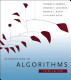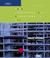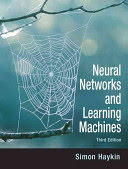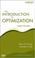### Advanced Algorithms and Data Structures

#### Course Description

Data structures: skip-lists, self-organizing lists, sparse tables, balanced trees (rotations in trees, AVL trees, RB trees), multiway trees, B-trees, trie. Selected topics on optimization: dynamic programming, introduction to genetic algorithms, introduction to neural networks (backpropagation algorithm), linear programming and simplex algorithm. Operations on sets. Selected graph algorithms: graph traversals, depth first search (DFS), breadth first search (BFS), shortest paths, cycle detection and connectivity, spanning trees, Eulerian graphs, Hamiltonian graphs, matching and assignment problems.

#### General Competencies

This course is a continuation of Algorithms and Data Structures undergraduate course and it is aimed to students wishing to widen and deepen the understanding of most important algorithms and data structures. Algorithms needed in various applications are presented, with the emphasis on understanding of their underlying logic and theoretical foundation. Specific program solutions are analyzed during laboratory exercises. By adopting the subject, the students will notably widen their competences and abilities to solve more complex problems that require correct selection and an efficient implementation of different algorithms.

#### Learning Outcomes

1. recognize and analyze the problem to be solved by computer
2. relate the specified problem to the one of the same kind or a similar known and already solved problem
3. select the best algorithm for a known problem
4. design one’s own algorithm for an unknown problem
5. assess the validity of one’s own solution, in the sense of algorithm convergence and complexity
6. compare and contrast own solution to possible alternatives
7. recommend the algorithm for an unknown problem on the basis of analysis of alternatives

#### Forms of Teaching

Lectures

Lectures are organized in 15 weeks, which accounts for two weeks for intermediate exams. Regularly, there is one lecture in a week and it lasts three hours in a block.

Exams

Exams are written and oral.

Exercises

Auditory exercises are held occasionally by volunteer students.

Laboratory Work

Obligatory, performed at home; assessed by assistants.

Consultations

By arrangement.

Seminars

By arrangement. Students can prepare short presentations of selected algorithms or their own solutions.

#### Grading Method

Continuous Assessment Exam
Type Threshold Percent of Grade Threshold Percent of Grade
Class participation 0 % 8 % 0 % 0 %
Mid Term Exam: Written 25 % 42 % 0 %
Final Exam: Written 30 % 50 %
Exam: Written 70 % 50 %
Exam: Oral 50 %

#### Week by Week Schedule

1. Introductory lesson. Data structures: skip-lists, self-organizing lists, sparse tables. Decission trees and sorting. Deletions in trees: deletion by merging, deletion by copying. Balanced trees – introduction. DSW algorithm.
2. Self-adjusting trees. AVL Trees. B-trees.
3. Multiway trees. B-trees. Red-black trees.
4. Structure Trie. Introduction to optimization. Genetic algorithms.
5. Genetic algorithms. Example of graph colouring, travelling salesman problem. Introduction to neural networks.
6. Neural networks.
7. Dynamic programming, example of the knapsack problem.
8. Intermediate exam.
9. Intermediate exam.
10. Linear programming and simplex algorithm.
11. Linear programming and simplex algorithm.
12. Backtracking. Problem of eight queens and the problem of maze and their comparison. An introduction to graph algorithms: graph traversals, depth first search (DFS), breadth first Search (BFS). Shortest paths (Dijkstra, Bellman-Ford).
13. Shortest paths (Warshall-Floyd-Ingerman algorithm). Spanning trees. Operations on sets (union-find) and graph connectivity. Eulerian graphs - introduction.
14. Fleury algorithm, Eulerization of a graph, the Chinese postman problem. Stating matching and assignment problems. Flow in networks (Ford-Fulkerson algorithm) with application to matching in a graph.
15. Hamiltonian graphs. Travelling salesman problem.

#### Study Programmes

##### University graduate
Computer Engineering (profile)
Theoretical Course (1. semester)
Computer Science (profile)
Theoretical Course (1. semester)
Information Processing (profile)
Theoretical Course (1. semester)
Software Engineering and Information Systems (profile)
Theoretical Course (1. semester)
Telecommunication and Informatics (profile)
Specialization Course (1. semester) (3. semester)

#### LiteratureCormen, Leiserson, Rivest, Stein (2009.), Introduction to algorithms, MIT PressAdam Drozdek (2004.), Data Structures and Algorithms in C++, Thomson Course TechnologySimon Haykin (2009.), Neural Networks and Learning Machines, Prentice Hall
D.Kalipć, V.Mornar (1996.), Operacijska istraživanja, ZuesE.K.P. Chong, S.H.Żak (2008.), An Introduction to Optimization, Wiley Interscience

#### General

ID 34395
Winter semester
5 ECTS
L1 English Level
L1 e-Learning
45 Lectures
0 Exercises
0 Laboratory exercises
0 Project laboratory

90 Excellent
75 Very Good
60 Good
50 Acceptable